## STAM101 :: Lecture 22 :: Strip plot design – layout – ANOVA Table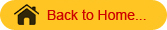Strip Plot Design

This design is also known as split block design. When there are two factors in an experiment and both the factors require large plot sizes it is difficult to carryout the experiment in split plot design. Also the precision for measuring the interaction effect between the two factors is higher than that for measuring the main effect of either one of the two factors. Strip plot design is suitable for such experiments.

In strip plot design each block or replication is divided into number of vertical and horizontal strips depending on the levels of the respective factors.

Replication 1                                                     Replication 2

a0            a2            a3            a1                                                  a3              a0            a2            a1

 b1 b0 b2
 b1 b2 b0

In this design there are plot sizes.

1. Vertical strip plot for the first factor – vertical factor
2. Horizontal strip plot for the second factor – horizontal factor
3. Interaction plot for the interaction between 2 factors

The vertical strip and the horizontal strip are always perpendicular to each other. The interaction plot is the smallest and provides information on the interaction of the 2 factors. Thus we say that interaction is tested with more precision in strip plot design.

# Analysis

The analysis is carried out in 3 parts.

1. Vertical strip analysis
2. Horizontal strip analysis
3. Interaction analysis

Suppose that A and B are the vertical and horizontal strips respectively. The following two way tables, viz., A X Rep table, B X Rep table and A X B table are formed. From A X Rep table, SS for Rep, A and Error (a) are computed. From B X Rep table, SS for B and Error (b) are computed. From A X B table, A X B SS is calculated.

When there are r replications,  a levels for factor A and b levels for factor B, then the ANOVA table is

 X d.f. SS MS F Replication (r-1) RSS RMS RMS/EMS (a) A (a-1) ASS AMS AMS/EMS (a) Error (a) (r-1) (a-1) ESS (a) EMS (a) B (b-1) BSS BMS BMS/EMS (b) Error (b) (r-1) (b-1) ESS (b) EMS (b) AB (a-1) (b-1) ABSS ABMS ABMS/EMS (c) Error (c) (r-1) (a-1) (b-1) E SS (c) EMS (c) Total               (rab – 1)                 TSS

Analysis
Arrange the results as follows:

 Treatment Combination Replication Total R1 R2 R3 … A0B0 a0b0 a0b0 a0b0 … T00 A0B1 a0b1 a0b1 a0b1 … T01 A0B2 a0b2 a0b2 a0b2 … T02 Sub Total A01 A02 A03 … T0 A1B0 a1b0 a1b0 a1b0 … T10 A1B1 a1b1 a1b1 a1b1 … T11 A1B2 a1b2 a1b2 a1b2 … T12 Sub Total A11 A12 A13 … T1 . . . . . . . . . . . . . . . . . . Total R1 R2 R3 … G.T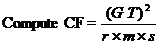TSS = [ (a0b0)2 + (a0b1)2+(a0b2)2+…]-CF

1. Vertical Strip Analysis

Form A x R Table and calculate RSS, ASS and Error(a) SS

 Treatment Replication Total R1 R2 R3 … A0 A01 A02 A03 … T0 A1 A11 A12 A13 … T1 A2 A21 A22 A23 … T2 . . . . . . . . . . . . . . . . . . Total R1 R2 R3 … GT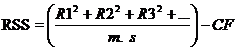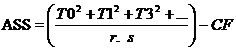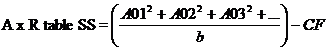Error (a) SS= A x R TSS-RASS-ASS.

1. Horizontal  Strip Analysis

Form B x R Table and calculate RSS, BSS and Error(b) SS

 Treatment Replication Total R1 R2 R3 … B0 B01 B02 B03 … T0 B1 B11 B12 B13 … T1 B2 B21 B22 B23 … T2 . . . . . . . . . . . . . . . . . . Total R1 R2 R3 … GT
1.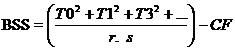2.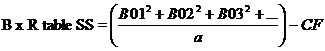3. Error (b) SS= B x R TSS-RSS-BSS

3) Interaction Analysis
Form A xB Table and calculate BSS, Ax B SSS and Error (b) SS

 Treatment Replication Total B0 B1 B2 … A0 T00 T01 T02 … T0 A1 T10 T11 T12 … T1 A2 T20 T21 T22 … T2 . . . . . . . . . . . . . . . . . . Total C0 C1 C2 … GT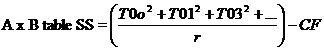ABSS= A x B Table SS – ASS- ABSS
Error (c) SS= TSS-ASS-BSS-ABSS –Error (a) SS.- –Error (a) SS
Then complete the ANOVA table.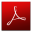Download this lecture as PDF here